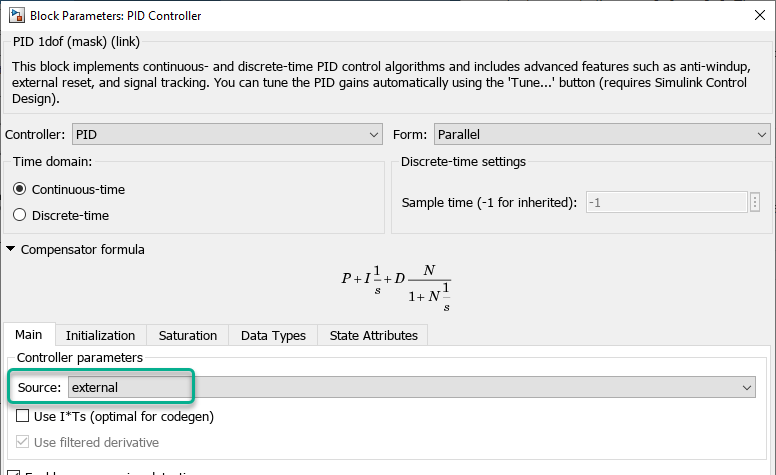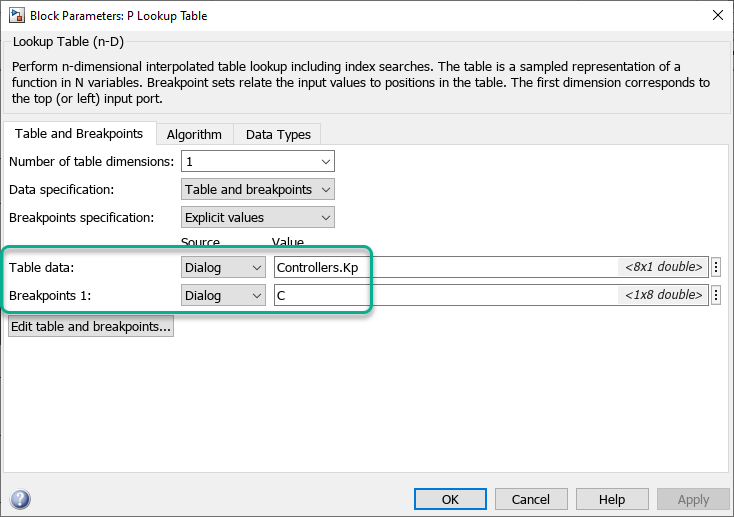# Implement Gain-Scheduled PID Controllers

This example shows how to implement gain-scheduled control in a Simulink® model using a family of PID controllers. The PID controllers are tuned for a series of steady-state operating points of the plant, which is highly nonlinear.

This example builds on the work done in Design Family of PID Controllers for Multiple Operating Points. In that example, the continuous stirred-tank reactor (CSTR) plant model is linearized at steady-state operating points that have output concentrations `C` = 2, 3, ..., 8, 9. The nonlinearity in the CSTR plant yields different linearized dynamics at different output concentrations. The example uses the `pidtune` function to generate and tune a separate PID controller for each output concentration.

You can expect each controller to perform well in a small operating range around its corresponding output concentration. This example shows how to use the PID Controller block to implement all of these controllers in a gain-scheduled configuration. In such a configuration, the PID gains change as the output concentration changes. This configuration ensures good PID control at any output concentration within the operating range of the control system.

Begin with the controllers generated in Design Family of PID Controllers for Multiple Operating Points. If these controllers are not already in the MATLAB® workspace, load them from the data file `PIDGainSchedExample.mat`.

`load PIDGainSchedExample`

This operation puts two variables in the MATLAB workspace, `Controllers` and `C`. The model array `Controllers` contains eight `pid` models, each tuned for one output concentration in the vector `C`.

To implement these controllers in a gain-scheduled configuration, create lookup tables that associate each output concentration with the corresponding set of PID gains. The Simulink model `PIDGainSchedCSTRExampleModel` contains such lookup tables, configured to provide gain-scheduled control for the CSTR plant. Open this model.

`open_system("PIDGainSchedCSTRExampleModel")`In this model, the PID Controller block is configured to have external input ports for the PID coefficients. Using external inputs allows the coefficients to vary as the output concentration varies. Open the block to examine the configuration.Setting the Source parameter to `external` enables the input ports for the coefficients.

The model uses a 1-D Lookup Table block for each of the PID coefficients. In general, for gain-scheduled PID control, use your scheduling variable as the lookup-table input, and the corresponding controller coefficient values as the output. In this example, the CSTR plant output concentration is the lookup table input, and the output is the PID coefficient corresponding to that concentration. To see how the lookup tables are configured, open the `P Lookup Table` block.The Table data parameter contains the array of proportional coefficients for each controller, `Controllers.Kp`. (For more information about the properties of the `pid` models in the `Controllers` array, see `pid`.) Each entry in this array corresponds to an entry in the array `C` that is entered in the Breakpoints 1 parameter. For concentration values that fall between entries in `C`, the P Lookup Table block performs linear interpolation to determine the value of the proportional coefficient. To set up lookup tables for the integral and derivative coefficients, configure the `I Lookup Table` and `D Lookup Table` blocks using `Controllers.Ki` and `Controllers.Kd`, respectively. For this example, this configuration is already done in the model.

The `pid` models in the `Controllers` array express the derivative filter coefficient as a time constant, `Controllers.Tf` (see the `pid` reference page for more information). However, the PID Controller block expresses the derivative filter coefficient as the inverse constant, `N`. Therefore, the `N Lookup Table` block must be configured to use the inverse of each value in `Controllers.Tf`. Open the `N Lookup Table` block to see the configuration.Simulate the model. The `Concentration Setpoint` block is configured to step through a sequence of setpoints that spans the operating range between `C` = 2 and `C` = 9 (shown in yellow on the scope). The simulation shows that the gain-scheduled configuration achieves good setpoint tracking across this range (blue on the scope).As was shown in Design Family of PID Controllers for Multiple Operating Points, the CSTR plant is unstable in the operating range between `C` = 4 and `C` = 7. The gain-scheduled PID controllers stabilize the plant and yield good setpoint tracking through the entire unstable region. To fully validate the control design against the nonlinear plant, apply a variety of setpoint test sequences that test the tracking performance for steps of different sizes and directions across the operating range. You can also compare the performance against a design without gain scheduling, by setting all entries in the `Controllers` array equal.